Courses

# 28 Year NEET Questions: Chemical Bonding And Molecular Structure- 3

## 30 Questions MCQ Test Chemistry 31 Years NEET Chapterwise Solved Papers | 28 Year NEET Questions: Chemical Bonding And Molecular Structure- 3

Description
This mock test of 28 Year NEET Questions: Chemical Bonding And Molecular Structure- 3 for NEET helps you for every NEET entrance exam. This contains 30 Multiple Choice Questions for NEET 28 Year NEET Questions: Chemical Bonding And Molecular Structure- 3 (mcq) to study with solutions a complete question bank. The solved questions answers in this 28 Year NEET Questions: Chemical Bonding And Molecular Structure- 3 quiz give you a good mix of easy questions and tough questions. NEET students definitely take this 28 Year NEET Questions: Chemical Bonding And Molecular Structure- 3 exercise for a better result in the exam. You can find other 28 Year NEET Questions: Chemical Bonding And Molecular Structure- 3 extra questions, long questions & short questions for NEET on EduRev as well by searching above.
QUESTION: 1

### The angle between the overlapping of one s-orbital and one p-orbital is 

Solution: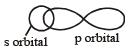The overlap between s- and p-orbitals occurs along internuclear axis and hence the angle is 180°.

QUESTION: 2

### Equilateral shape has 

Solution:

Equilateral or triangular planar shape involves sp2 hybridization.

QUESTION: 3

### Which of the following molecule does not have a linear arrangement of atoms ? 

Solution:

For linear arrangement of atoms the hybridisation should be sp(linear shape, 180° angle). Only H2S has sp3-hybridization and hence has angular shape while C2H2, BeH2 and CO2 all involve sp - hybridization and hence have linear arrangement of atoms.

QUESTION: 4

Which of the following does not apply to metallic bond ?

Solution:

In metallic bonds each ion is surrounded by equal no. of oppositely charged ions hence have electrostatic attraction on all sides and hence do not have directional characteristics.

QUESTION: 5

In which one of the following molecules the central atom said to adopt sp2 hybridization?

Solution:
QUESTION: 6

H2O has a non zero dipole moment while BeF2 has zero dipole moment because 

Solution:

BeF2 is linear and hence has zero dipole moment while H2O, being a bent molecule, has a finite or non-zero dipole moment.

QUESTION: 7

Among LiCl, BeCl2 BCl3 and CCl4, the covalent bond character follows the order 

Solution:

As we move in period from Li → Be → B → C, the electronegativity (EN)  increases and hence the EN difference between the element and Cl decreases and accordingly the covalent character increases Thus option (c) i.e., LiCl < BeCl2 < BCl3< CCl4 is correct.

QUESTION: 8

Which statement is NOT correct ? 

Solution:

A σ - bond is stronger than a σ -bond hence option (a) is not correct. Sigma (σ) bonds are formed by head on overlap of  unhybridised s–s, p–p or s–p orbitals and hybridised orbitals (sp, sp2, sp3, sp3d and sp3d2) hence σ bonds  are strong bonds. Where as Pi (π)-bonds are formed by side ways overlap of unhybridised p- and d orbitals-hence σ bonds are weak bonds.

QUESTION: 9

Which one shows the strongest hydrogen bonding?

Solution:

Among the F, O, and N, Fluorine has a higher value of electronegativity, this is the reason that fluorine can form the strongest hydrogen bond with the H atom of another molecule.

Just take an idea from the value of the energy of hydrogen bond that is formed with HF, H2O, and NH3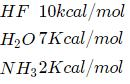means to break the hydrogen of one mole HF molecules, 10Kcal energy is required.

QUESTION: 10

Linear combination of two hybridized orbitals belonging to two atoms and each having one electron leads to a 

Solution:

Linear combination of two hybridized orbitals leads to the formation of sigma bond.

QUESTION: 11

Which one of the following formulae does not correctly represent the bonding capacities of the two atoms involved ? 

Solution: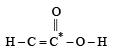The  star marked carbon has a valency of 5 and hence this formula is not correct.

QUESTION: 12

In compound X, all the bond angles are exactly 109°28; X is 

Solution:

CCl4 has sp3 hybridisation , tetrahedral geometry and all bond angles of 109° 28'.

QUESTION: 13

Which of the following bonds will be most polar?

Solution:

Polarity of the bond depends upon the electronegativity difference of the two atoms forming the bond. Greater the electronegativity difference, more is the polarity of the bond.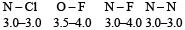As the electronegativity difference between N and F is maximum hence this bond is most polar.

QUESTION: 14

Which one of the following has the shortest carbon carbon bond length ? 

Solution:

The bond length decreases in the order sp3 > sp2 > sp.
Because of the triple bond, the carbon-carbon bond distance in ethyne is shortest.

QUESTION: 15

Which structure is linear ? 

Solution:

CO2 has sp-hybridization and is linear. SO2 and CO2-3 are planar (sp2) while SO2-is tetrahedral (sp3).

QUESTION: 16

Strongest hydrogen bond is shown by 

Solution:

H – F shows strongest H-bonds.  Linear combination of two hybridized orbitals leads to the formation of sigma bond.

QUESTION: 17

Which of the following statements is not correct ? 

Solution:

Sigma bond is stronger than π-bond. The electrons in the π bond are loosely held. The bond is easily broken and is more reactive than σ-bond. Energy released during sigma bond formation is always more than π bond because of greater  extent of overlapping.

QUESTION: 18

Among the following which compound will show the highest lattice energy ? 

Solution:

For compounds containing ion s of same charge, lattice energy increases as the size the ions decrease. Thus, NaF has highest lattice energy. The size of cation is in the order Na+ < K+ < Rb+ < Cs+

QUESTION: 19

Which one of the following is the correct order of interactions ? 

Solution:

The strength of the interactions follows the order vander Waal’s < hydrogen – bonding < dipole-dipole < covalent.

QUESTION: 20

Strongest bond is in between 

Solution:

According to Fajan rules, ionic character increases with increase in size of the cation and decrease in size of the anion. Thus, CsF has higher ionic character than NaCl and hence bond in CsF is stronger than in NaCl.

QUESTION: 21

Mark the incorrect statement in the following 

Solution:

The removal of an electron from a diatomic molecule may increase the bond order as in the conversion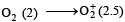or decrease the bond order as in the conversion,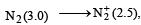As a result, the bond energy may increase or decrease. thus, statement (b) is incorrect.

QUESTION: 22

The weakest among the following types of bonds is

Solution:

H-bond is the weakest.

QUESTION: 23

Which of the following does not have a tetrahedral structure ? 

Solution:

BH3 has sp2 hybridization and hence does not have tetrahedral structure while all others have tetrahedral structures.

QUESTION: 24

Which of the following pairs will form the most stable ionic bond ? 

Solution:

The stability of the ionic bond depends upon the lattice energy which is expected to be more between Mg and F due to +2 charge on Mg atom.

QUESTION: 25

Among the following orbital bonds, the angle is minimum between 

Solution:

The angle between the bonds formed by px and py orbitals is the minimum i.e. 90°

QUESTION: 26

Linus Pauling received the Nobel Prize for his work on 

Solution:

Chemical bonds.

QUESTION: 27

The boiling point of p-nitrophenol is higher than that of o-nitrophenol because 

Solution:

The b.p. of p-nitrophenol is higher than that of o-nitrophenol because in p-nitrophenol there is intermolecular H-bonding but in onitrophenol it is intramolecular H-bonding.

QUESTION: 28

The distance between the two adjacent carbon atoms is largest in 

Solution:

The C–C bond distance decreases as the multiplicity of the bond increases. Thus, bond distance decreases in the order: butane (1.54 Å) > benzene (1.39 Å) > ethene (1.34) Å > ethyne (1.20 Å). Thus in butane, C – C bond distance is the largest.

QUESTION: 29

Which of the following species is paramagnetic?

Solution:

Paramagnetism is caused by the presence of atoms, ions or molecules with unpaired electrons. In NO the presence of unpaired electron is clear. Therefore it is paramagnetic.

QUESTION: 30

The BCl3 is a planar molecule whereas NCl3 is pyramidal because 

Solution:

As there is no lone pair on boron in BCl3 therefore no repulsion takes place. But there is a lone pair on nitrogen in NCl3. Therefore repulsion takes place. Thus BCl3 is planar molecule but NCl3 is a pyramidal molecule.